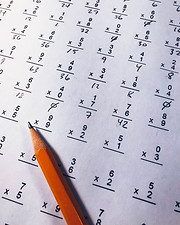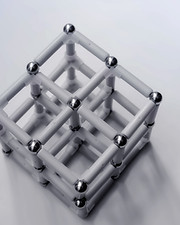top of page

#### MATH

##### Math Registration

Registration is open for our In-Person and Online Math, Logic and Lego classes!

##### Instructor(s)
• Group lessons: In our online and in-person math classes, we help the students develop logical thinking routines, to prepare them for AMC, Math Kangaroo, MOEMS and other math competitions.

• Math Competition Teams: We organize math competition teams for Math Kangaroo, MOEMS and other math contests. Instructions and training are provided to help the students be well prepared and build confidence in the math competitions.

• Assessments: Ongoing assessments are arranged via monthly tests and weekly homework, to make sure the students master the contents of the courses and make good progress in their learning journey.Miss Eralin

Math TeacherMiss King

Math TeacherDr Qin

Math TeacherMiss Chien
Math TeacherGT, Logic & Math

This class builds and develops the mathematical thinking ability of kindergarten students

IN-PERSONLego & Engineering

This class engages kids using LEGO® bricks and technology to help them explore science, technology, engineering and math concepts.

IN-PERSONIN-PERSONAMC 8

This is an AMC 8 Competition preparation course for 5th grade to 7th grade students.

ONLINEAMC 10

This is an AMC 8 Competition preparation course for 5th grade to 7th grade students.

ONLINE

ONLINE##### Overview

This class engages kids using LEGO® bricks and technology to help them explore science, technology, engineering and math concepts.

##### Class Schedule
1. Carousel

2. Scarecrow

3. Cuckoo Clock

4. Lift Bed

5. Train

6. Sweeper

7. Helicopter

8. Percussion Instruments

9. Wipers

10. Walking Cow

11. Crossbow

12. Egg Beater

13. Well

##### Class Schedule
• IN-PERSON: Mondays, 3:30 pm - 4:30 pm

• IN-PERSON: Mondays, 4:30 pm - 5:30 pm##### Overview

This course covers the content from GT tests and private school entry exams. This will help improve students' concentration, memory and critical thinking abilities.

##### Objectives
• Number & picture analogies

• Number puzzles

• Arithmetic reasoning

• Number series

• Spatial visualization

• Pattern completion

• Figural series

• Following directions

• Picture concepts

• Oral vocabulary

• Aural reasoning

• Verbal classification

• Verbal reasoning

• Relational concepts

##### Class Schedule
• IN-PERSON: Thursdays, 3:30pm - 4:30pm##### Overview

Math Olympiad aims to discover the “fun” in mathematics and enhance children’s logical reasoning ability by focusing on the exploration of different problem-solving strategies. The course uses Beast Academy as the main content and adds Gao Si Math (Chinese Math Olympiad Textbook) and basic practices to complete the math competition learning system.

##### Objectives

• Pirate Symbols and regrouping, convert to numbers

• Breaking and Regrouping

• Adding and Subtracting 1, 10, and 100

• The Number Line

• Comparing

• Ordering (missing digits)

• Sum with regrouping

• Addition strategies: counting up and compensation

• Place value subtraction strategy

• Subtraction strategy: counting up, subtract then add

• Subtraction strategy: changing difference, changing order

• Expression and parentheses

• Symbols (An Intro to Variables)

• Simplify expression

• Strategy: Guessing and Checking / Working Backwards

• Strategy: Draw a Picture & Extra Information

• Puzzles comprehensive practice

• Units, Changing units & Mixed measures

• Addition and Subtraction with units

• Rearranging & Cancelling

• All at Once & Parentheses

• Skip counting patterns

• Problem Solving with Parity (Inside/Outside, coin, checkboards)

• Counting Up & Counting by 10’s, 100’s, 1,000’s

• Comparing and Ordering

• Estimation

• Stacking addition & subtraction, checking results

• Stacking puzzles

• Organization & Sorting

• Solve a Simpler Problem

• Problem Solving Puzzles

• Directions and Mazes

• Queues and Patterns

• Symmetry and Overlapping Problems

• Counting 2D and 3D Shapes

• 3D Shapes, Different Views and Nets

• Concept of Possibility and Split Problems

• Multi-Step Word Problems & Logical Reasoning

• Match Puzzles and Giving Problems

##### Class Schedule
• IN-PERSON: Fridays, 3:30 pm - 4:30 pm (Prep)

• IN-PERSON: Fridays, 4:30 pm - 5:30 pm (Level 1)

• ONLINE: Mondays, 6:00 pm - 7:00 pm (Prep)

• ONLINE: Mondays, 7:00pm - 8:00 pm (Level 1)##### Overview

Math Olympiad aims to discover the “fun” in mathematics and enhance children’s logical reasoning ability by focusing on the exploration of different problem-solving strategies. The course uses Beast Academy as the main content and adds Gao Si Math (Chinese Math Olympiad Textbook) and basic practices to complete the math competition learning system.

##### Objectives

• Classifying Angles & Triangles

• Counting Shapes & Puzzles

• Patterns & Commutativity

• Starting at Different Numbers

• Perimeter & Triangle Inequality

• Perimeter of Rectilinear Shapes

• Area of regular & rectilinear shapes

• Multiplication Strategies: *4/8, *5, *10

• Rearranging Products

• Multiplication Word Problems

• Visual Models of Computation Strategies

• Perimeter and Area

• Multiplying Nearby Numbers

• The Area Model

• Distributing and Factoring

• Variables, Evaluating and Simplifying Expressions

• Solving Equations (Guess-and-Check & “Balance Scale” Methods)

• Use Varialbes for Patterns & Geometry

• Word Problems and Translating

• Relating Multiplication and Division

• Long Division and Remainders

• Division Word Problems

• Units, Addition & Subtraction, Unit Conversions

• Types of Measurements

• Estimation and Word Problems

• Fractions & Graphs (Number Line, Parts of Whole)

• Mixed Numbers and Equivalent Fractions

• Comparing and Ordering

• Goals of Estimation & Rounding

• Over- and Under-estimating, word problems

• Estimation Word Problems

• Area of Rectangles and Rectilinear Shapes

• Area of Triangles

• Area with Different Units & Word Problems

• Age & Cycle Problems

• Difference & Multiple Problems

• Chicken & Rabbit Problems， Permutations

• Odd and Even Problems, Steps

• Shapes and Counting Shapes

• Shape Change and Puzzle

• Time Problem

• Queues and Counting List

##### Class Schedule
• ONLINE: Tuesdays, 5:30 pm - 6:30 pm##### Overview

Math Olympiad aims to discover the “fun” in mathematics and enhance children’s logical reasoning ability by focusing on the exploration of different problem-solving strategies. The course uses Beast Academy as the main content and adds Gao Si Math (Chinese Math Olympiad Textbook) and basic practices to complete the math competition learning system.

##### Objectives

• Angle Measurement

• Classifying Shapes

• Symmetry

• The Distributive Property & Area Model

• The Partial Products Algorithm

• Estimation and Patterns

• Exponent Basics

• Manipulating Expressions with Exponents

• Exponent Expression Puzzle

• Counting List

• Possibilities

• Venn Diagrams

• Arrangement (Count Possibilities)

• Division Strategy (split a quotient into parts)

• Divisibility

• Logical Reasoning

• Logic Puzzle

• Primes and Composites

• Divisibility

• Prime Factorization

• Computation Strategies (Regrouping, subtract then add, make easy sums)

• Subtraction

• Patterns

• Comprehensive Integer Calculation

• Multiplication (meaning and computation)

• Division (meaning and computation)

• Mixed numbers and Geometry

• Comparing and Ordering

• Converting Between Decimals and Fractions

• Counting Review and Probability Introduction

• Random and Coins

• Compliments and Pairs

• Independent and Dependent

• Difference & Multiple Problems

• Cycle Problems

• Advanced Word Problem (Chicken and Rabbit)

• Grouping and Hypothesis Strategies

• Arrays and Matrix

• Advanced Profit and Loss Problem

• Advanced Word Problem (Drawer Theory & Unit)

##### Class Schedule
• Online: Wednesdays, 6:30 pm - 7:30 pm##### Overview

Math Olympiad aims to discover the “fun” in mathematics and enhance children’s logical reasoning ability by focusing on the exploration of different problem-solving strategies. The course uses Beast Academy as the main content and adds Gao Si Math (Chinese Math Olympiad Textbook) and basic practices to complete the math competition learning system.

##### Objectives

• Venn Diagrams & Factorials

• Addition and Subtraction (Unlike Denominators)

• Multiplication & Division

• Simplifying

• Arithmetic Sequences

• Arithmetic Series

• Geometric and Other Sequences

• Using Ratios

• Proportional Reasoning

• Rates

• Multiplying Decimals by Powers of 10

• Converting Fractions to Decimals

• Divison

• Conversions

• Percent of a Number

• Equations, Percent Change

• Comparing

• Tricky Square Roots (Factoring)

• The Pythagorean Theorem

• Multiplying and Dividing Powers

• Zero and Negative Exponents

• Raising a Power to a Power

##### Class Schedule
• Online: Fridays, 6:00 pm - 7:00 pm EST##### Overview

The AMC 8 is a 25-question, 40-minute, multiple choice examination in middle school mathematics designed to promote the development of problem-solving skills. The AMC 8 provides an opportunity for middle school students to develop positive attitudes towards analytical thinking and mathematics that can assist in future careers. Students apply classroom skills to unique problem-solving challenges in a low-stress and friendly environment.

The material covered on the AMC 8 includes topics from a typical middle school mathematics curriculum. Possible topics include but are not limited to: counting and probability, estimation, proportional reasoning, elementary geometry including the Pythagorean Theorem, spatial visualization, everyday applications, and reading and interpreting graphs and tables. In addition some of the later questions may involve linear or quadratic functions and equations, coordinate geometry, and other topics traditionally covered in a beginning algebra course.

##### Class Schedule
• Online Mock Test (40min) + Question Solving & Analyzing: 12/22/2022, 01/09/2023, 01/12/2023##### Overview

This is the continuation course to the AMC8 course, and the goal is to bridge the gap between AMC8 and AMC10. Students planning to get a competitive score in AMC8 or to participate in AMC10 for the first time to test water are all good candidates for this course. More advanced math knowledge and problem solving skills will be covered. Problems in this course are becoming more challenging, and hopefully students will enjoy cracking out more impossible problems.

##### Objectives
• Absolute values I (+)

• Absolute values II (+)

• Floor and ceiling (IA 20.3, MA 16.4-16.5)

• Exponents and radicals (IA 1.6-1.8)

• Expressions (IA 2)

• Advanced linear equations (IA3.2(3.11, 3.2.3-3.2.4), 3.5, Challenge)

• Multivariate expressions (IA 4)

• Advanced multivariate linear equations (IA5.3(5.3.3, 5.3.4), 5.5-5.6)

• Ratio and Percent(IA 6.1.5, 6.2-6.4)

• Percentage and Proportion (IA 6.5, 7.1-7.3)

• Rate (IA 7.4, Challenge)

• Cartesian plane, points and lines (IA 8.1-8.3)

• Line Equations (IA 8.4-8.5)

• Inequalities(IA 9.1-9.2)

• Inequalities and linear optimization(IA 9.3-9.5)

• Angles (IG 2)

• Congruent Triangles (IG 3.1-3.5, +)

• Perimeter and Area (IG 4, challenge)

• Intercept Theorem (+)

• Similar Triangles I(IG 5.1-5.5)

• Similar Triangles II (+)

• Right triangles selected (IG 6.1-6.5, challenge)

• Special parts of a triangle (Intro + IG 7.6, challenge)

• Polygons (IG 9)

• Primes, Composites and Divisors (+)

• Prime factorization and divisors (IN 4-5)

• Special numbers and algebra with Integers (IN 6-7)

• Base number (IN 8)

• Base number arithmetic(IN 9)

• Counting is arithmetic (don’t memorize) (IC 1)

• Casework, complementary, construction and restriction (IC2)

• Overcounting (IC 3)

• Combinations( IC 4)

• Combinations (IC 5)

##### Schedule
• ONLINE: Mondays & Thursdays, 7:00 pm - 8:30 pm EST (From 1/9/2023 –  6/15/2022 (no class during spring break week)

bottom of page